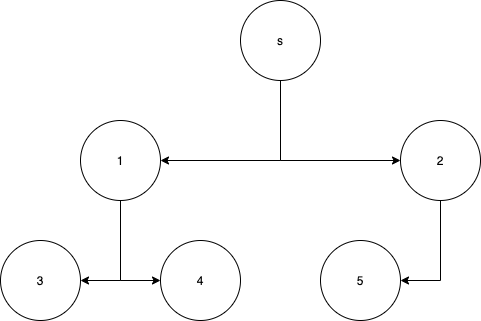1. Create a queue, add the root node.
2. While the queue has nodes, dequeue one.
3. Visit the node.
4. Add its children to the queue.
5. Repeat.

## Depth-First Search (DFS)

1. Create a stack, add the root node.
2. While the stack has nodes, pop one.
3. Visit the node.
4. Add its children to the stack.
5. Repeat until the stack is empty.

## Simplify

The real difference is only how we select the next node. Here’s a generic implementation common to both.

``````const search = nextNode => (root, visit) => {
let list = [root]
while (list.length) {
const node = nextNode(list)
visit(node)
list = list.concat(node.children)
}
}
``````

That’s it. If you can remember this, you’ve got them both down. Of course, there are complexities like cycles in graphs and inorder/postorder/preorder, but this is the basic concept.